• python 点云从相机坐标系到世界坐标系/ 世界坐标系到相机坐标系下的变换
千次阅读
2022-04-30 10:01:14

问题:

已知相机外参 extr 和 相机坐标系下的点云 pc 求解世界坐标系下的 点云 pc

已知相机外参 extr 和 世界坐标系下的点云 pc 求解相机坐标系下的 点云 pc

notes:

1. 一些经验之谈: 一般世界坐标系到相机坐标系的变换会发生在模拟器中(典型如pybullet, unity等), 因为模拟器中的世界坐标系原点坐标是(0, 0, 0). 但真机上使用该变换就需要采用相机标定以确定相机外参

2. 相机外参 extr: 不同模拟器有不同的表示形式 一般采用 extr的shape为(4, 4) 的矩阵来描述 (此时 相机的旋转R = extr[:3, :3], 相机的平移T = extr[:3, 3]), pybullet 中的extr采用 四元数 Q + 平移 T 的方式 描述 shape 为 (1, 7), 此时 相机的旋转 Q = extr[:, :4], 相机的平移 T = extr[:, 4:]

3. 上述表达中缩写的全称 extr --> extrinsic (外参),   pc -- > point cloud (点云)

4. 一些人会混淆 点云 和 世界坐标系 这两个概念 (而正确的认知应是世界坐标系下的点云, 与相机坐标系下的点云), 因为他们可能需要完成一些任务, 典型如测像素中两点对应真实世界中的两点的距离, 会出现一种思想:  世界中的两点距离 一定是需要"世界"坐标系的(二者正好均含世界二字).然而, 如果仅是相机测距的话仅需要在相机坐标系下的点云计算即可, 见python 深度图转点云_tycoer的博客-CSDN博客_深度图像转点云python 中的相机测距部分

import numpy as np
from scipy.spatial.transform import Rotation

def pc_cam_to_pc_world(pc, extrinsic):
# extrinsic 中旋转的表达形式为旋转矩阵
# pc shape (n , 3)
# extrinsic shape (4, 4)
extr_inv = np.linalg.inv(extrinsic)  # 求逆
R = extr_inv[:3, :3]
T = extr_inv[:3, 3]
pc = (R @ pc.T).T + T # 注意 R 需要左乘, 右乘会得到错误结果
return pc

def pc_world_to_pc_cam(pc, extrinsic):
R = extrinsic[:3, :3]
T = extrinsic[:3, 3]
pc = (R @ pc.T).T + T
return pc

if __name__ == '__main__':
# 造数据
np.random.seed(0)
extr = np.eye(4)
R = np.random.rand(3, 3)
# # 如果旋转部分采用了四元数
# from scipy.spatial.transform import Rotation
# Q = np.random.rand(1, 4)
# R = Rotation.from_quat(Q).as_matrix()

T = np.random.rand(3, 1)
extr[:3, :3] = R
extr[:3, 3:] = T

pc_cam = np.random.rand(1000, 3)
pc_world = pc_cam_to_pc_world(pc_cam, extr)
# 可以看到 pc_cam 和 pc_cam_val 的 值相同
pc_cam_val = pc_world_to_pc_cam(pc_world, extr)计算机视觉
更多相关内容
• ## 相机坐标系

千次阅读 2021-10-27 09:12:28
四个坐标系分别为：世界坐标系，相机坐标系，图像坐标系，像素坐标系 世界坐标系（world coordinate），也称为测量坐标系，是一个三维直角坐标系，以其为基准可以描述相机和待测物体的空间位置 相机坐标系{camera}...

### 相机坐标系

• 物距：被拍摄物体到凸透镜的距离
• 像距：成像平面到凸透镜的距离
• 焦距：凸透镜中心到焦点的距离

四个坐标系分别为：世界坐标系，相机坐标系，图像坐标系，像素坐标系

• 世界坐标系（world coordinate），也称为测量坐标系，是一个三维直角坐标系，以其为基准可以描述相机和待测物体的空间位置
• 相机坐标系{camera}：坐标原点为相机的光心位置，X 轴和Y 轴分别平行于图像坐标系的X轴和 Y 轴，Z轴为相机的光轴
• 图像坐标系：像素坐标系和图像坐标系都在成像平面上，只是各自的原点和度量单位不一样
• 像素坐标系：就是看到相机拍出来保存下来的图像。一般情况下相机坐标系光轴都在相机中心，所以成像的图像坐标系原点也在中心，然后人为转换到左上角原点的像素坐标系上

## 像素坐标系到图像坐标系

u-v坐标系表示看到相机保存下来的照片，x-y坐标系是成像的位置坐标系，可以认为是传感器实际成像区域，从图中位置可以看出x-y坐标系原点在u-v坐标系（u0,v0）上，因此可得变换关系：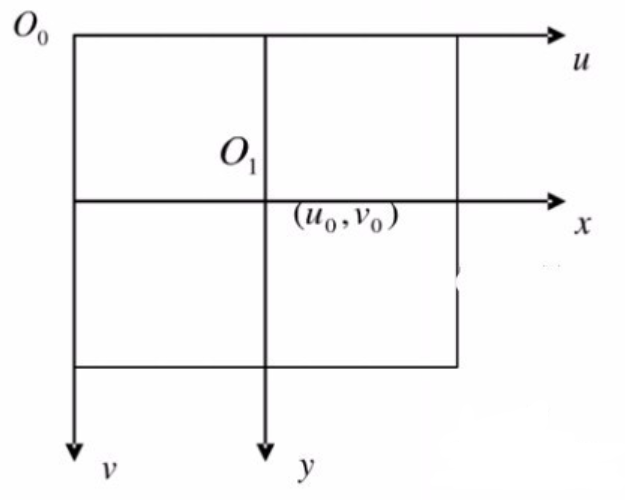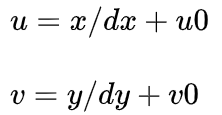d x ， d y dx，dy 为相机的固有参数，针对某一个型号传感器来说是固定的，分别表示在x，y方向上每个像素位置占多少毫米（mm/pixel），即 1 p i x e l = d x m m 1pixel=dx mm u 0 ， v 0 u_0，v_0 一般指图像上的中心位置，可能有一点点偏差。转换成齐次坐标形式就是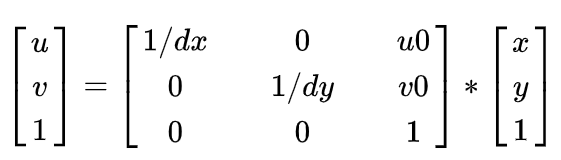## 图像坐标系到相机坐标系

根据针孔相机原理，相机坐标系上物体在图像坐标系上成立倒像，依据三角形相似可以得出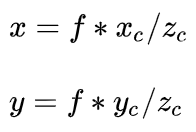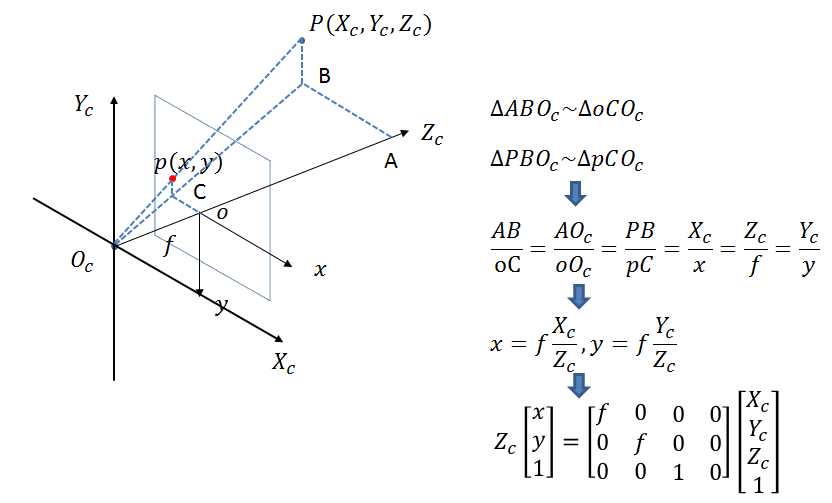转换为矩阵形式：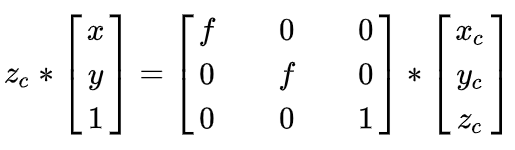其中Zc是相机坐标系某点所在的深度值。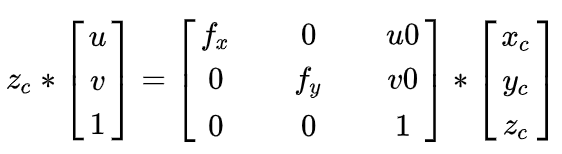其中 f f 为焦距， f = ∣ o − o c ∣ ， f x = f / d x ， f y = f / d y f=|o-o_c|，f_x=f/dx，f_y=f/dy ，中间矩阵称为相机的内参，一般认为是相机的固定参数。

## 世界坐标系和相机坐标系的转换

外部世界是一个大坐标系，相机在这个坐标系上一个点，相机前面有另外一个点，这个点假设在相机坐标系上的坐标是 （ x c , y c , z c ） （x_c, y_c, z_c） ，在世界坐标系上的坐标是 （ x w , y w , z w ） （x_w, y_w, z_w） ，想想一下上下左右前后的移动相机，就可以移动到这个点在两个坐标系中坐标一样，称为刚性变换。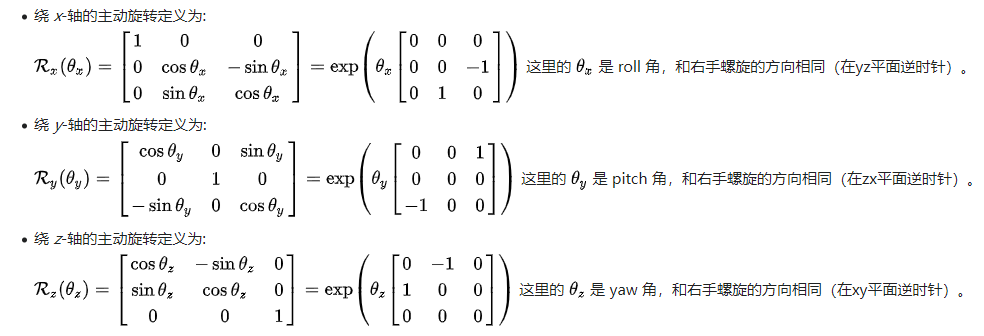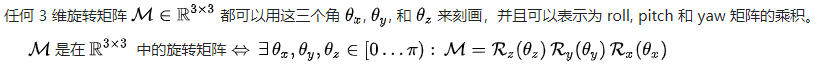公式右侧矩阵分别记为旋转矩阵 R R ，平移矩阵 T T ，成为相机的外参。用于多个传感器之间的标定。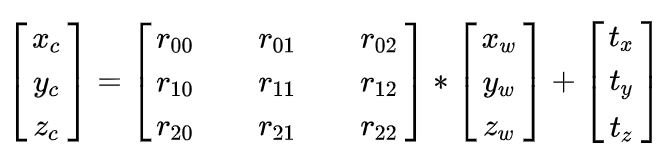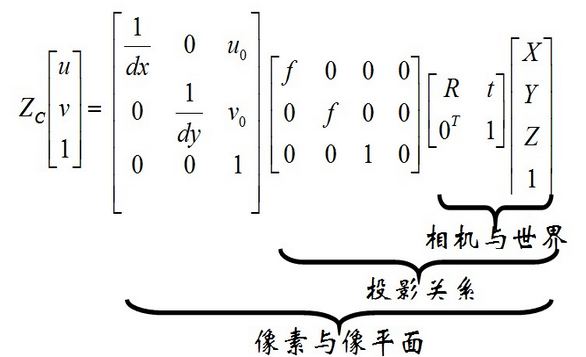## 添加畸变

径向畸变：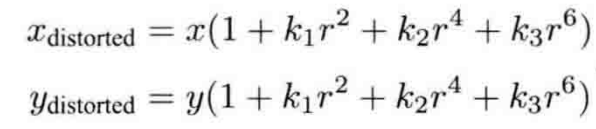切向畸变：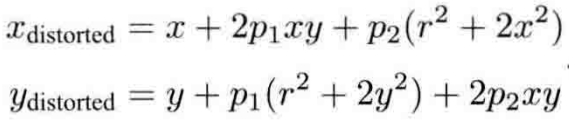对于相机坐标系中的一点P，可以通过5个畸变系数找到这个点在像素平面上的正确位置：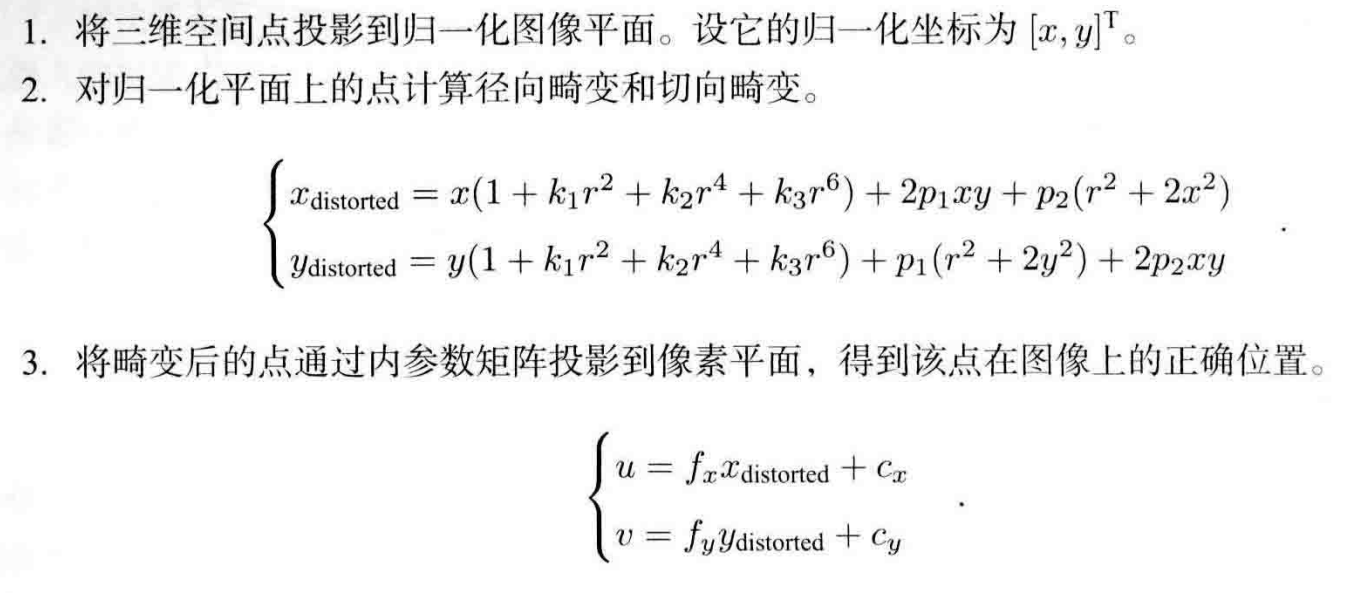python代码，从雷达到像素

# lidar -- camera -- pixel
param['rotate_vec'] = np.mat([1.5131235448660183, -1.504080508615343, 1.0724538041051752])
param['trans_vec'] = np.mat([-219.992648, 164.662458, 222.462379])
param['in_param'] = np.mat([[1152.85, 0, 957.033], [0, 1147.63, 529.578], [0, 0, 1.0]])
param['dist_vec'] = np.mat([-0.3272, 0.0985, 0.000015, 0.00049, 0])

camera_res = (np.dot(rot_mat, (np.array(p) * 10).reshape(-1, 1)) + np.array(param["trans_vec"]).T).squeeze()
camera_res = camera_res / camera_res
r = math.sqrt(camera_res ** 2 + camera_res ** 2)
tem_cam_x = camera_res * (1 + k1 * math.pow(r, 2) + k2 * math.pow(r, 4) + k3 * math.pow(r, 6))
tem_cam_y = camera_res * (1 + k1 * math.pow(r, 2) + k2 * math.pow(r, 4) + k3 * math.pow(r, 6))
tem_cam_x = tem_cam_x + 2 * p1 * camera_res * camera_res + p2 * (r ** 2 + 2 * camera_res ** 2)
tem_cam_y = tem_cam_y + p1 * (r ** 2 + 2 * camera_res ** 2) + 2 * p2 * camera_res * camera_res
camera_res = tem_cam_x
camera_res = tem_cam_y
img_x, img_y, _ = np.dot(np.array(param['in_param']), camera_res)

展开全文计算机视觉 opencv 图像处理
• 像素坐标系与图像坐标系 图像坐标系与相机坐标系 相机坐标系与世界坐标系
• ## 坐标系示意图

•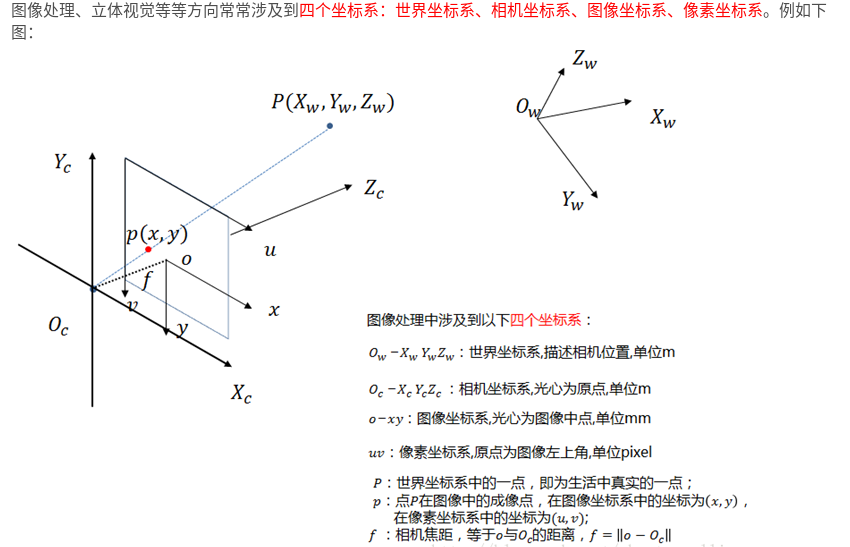• ## 像素坐标系与图像坐标系

• 像素坐标轴为uv，图像坐标轴为xy
• 参数为：dx, dy, u0, v0
•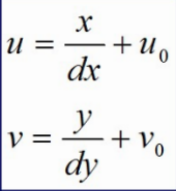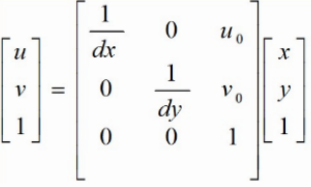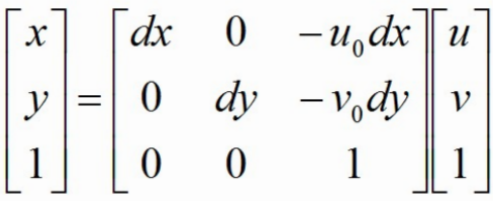• ## 图像坐标系与相机坐标系

•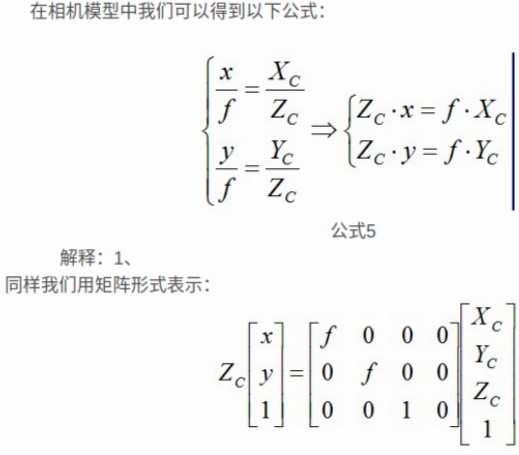• ## 相机坐标系与世界坐标系

•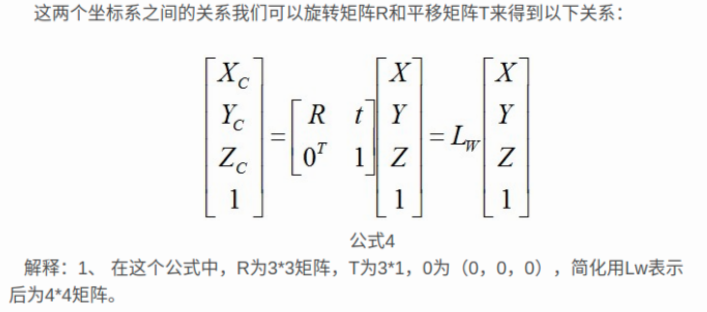• 旋转矩阵R
•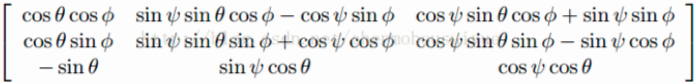• ## 像素坐标系映射到世界坐标系

•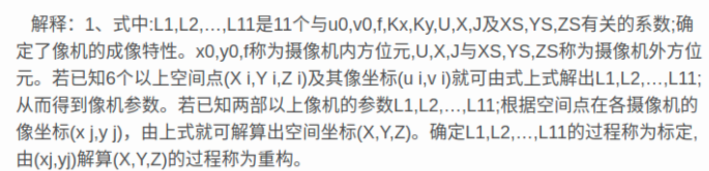•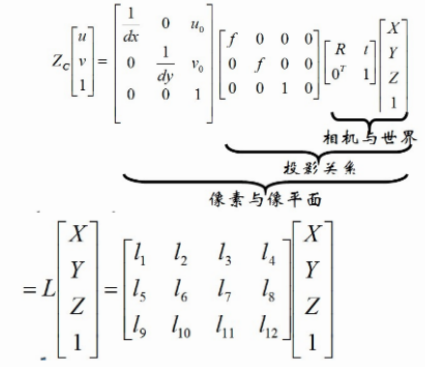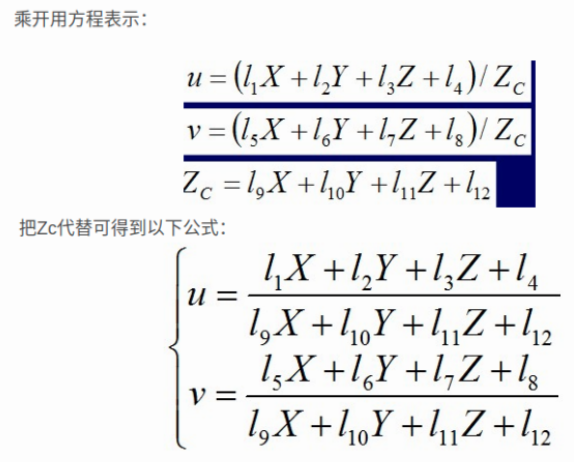• ## 各坐标系转换代码实现

• import sys
import os
sys.path.append(os.getcwd())
import numpy as np

camera_intrinsic = {
# R，旋转矩阵
"R": [[-0.91536173, 0.40180837, 0.02574754],
[0.05154812, 0.18037357, -0.98224649],
[-0.39931903, -0.89778361, -0.18581953]],
# t，平移向量
"T": [1841.10702775, 4955.28462345, 1563.4453959],
# 焦距，f/dx, f/dy
"f": [1145.04940459, 1143.78109572],
# principal point，主点，主轴与像平面的交点
"c": [512.54150496, 515.45148698]

}

class CoordinateConvert:
@staticmethod
def convert_wc_to_cc(joint_world):  # joint_world: n*3
"""
世界坐标系 -> 相机坐标系: R * pt + T
:return:
"""
joint_world = np.asarray(joint_world)
R = np.asarray(camera_intrinsic["R"])
T = np.asarray(camera_intrinsic["T"])
joint_num = len(joint_world)
# 世界坐标系 -> 相机坐标系
# [R|t] world coords -> camera coords
joint_cam = np.zeros((joint_num, 3))  # joint camera
for i in range(joint_num):  # joint i
joint_cam[i] = np.dot(R, joint_world[i]) + T  # R * pt + T
return joint_cam

@staticmethod
def __cam2pixel(cam_coord, f, c):
"""
相机坐标系 -> 像素坐标系: (f / dx) * (X / Z) = f * (X / Z) / dx
cx,ppx=260.166; cy,ppy=205.197; fx=367.535; fy=367.535
将从3D(X,Y,Z)映射到2D像素坐标P(u,v)计算公式为：
u = X * fx / Z + cx
v = Y * fy / Z + cy
D(v,u) = Z / Alpha
=====================================================
camera_matrix = [[428.30114, 0.,   316.41648],
[   0.,    427.00564, 218.34591],
[   0.,      0.,    1.]])
fx = camera_intrinsic[0, 0]
fy = camera_intrinsic[1, 1]
cx = camera_intrinsic[0, 2]
cy = camera_intrinsic[1, 2]
=====================================================
:param cam_coord:
:param f: [fx,fy]
:param c: [cx,cy]
:return:
"""
# 等价于：(f / dx) * (X / Z) = f * (X / Z) / dx
# 三角变换， / dx, + center_x
u = cam_coord[..., 0] / cam_coord[..., 2] * f + c
v = cam_coord[..., 1] / cam_coord[..., 2] * f + c
d = cam_coord[..., 2]
return u, v, d

@staticmethod
def convert_cc_to_ic(joint_cam):
"""
相机坐标系 -> 像素坐标系
:param joint_cam:
:return:
"""
# 相机坐标系 -> 像素坐标系，并 get relative depth
# Subtract center depth
# 选择 Pelvis骨盆 所在位置作为相机中心，后面用之求relative depth
root_idx = 0
center_cam = joint_cam[root_idx]  # (x,y,z) mm
joint_num = len(joint_cam)
f = camera_intrinsic["f"]
c = camera_intrinsic["c"]
# joint image，像素坐标系，Depth 为相对深度 mm
joint_img = np.zeros((joint_num, 3))
joint_img[:, 0], joint_img[:, 1], joint_img[:, 2] = CoordinateConvert.__cam2pixel(joint_cam, f, c)  # x,y
joint_img[:, 2] = joint_img[:, 2] - center_cam  # z: 相对图片某个点的深度信息
return joint_img

if __name__ == "__main__":
joint_world = [[-91.679, 154.404, 907.261],
[-223.23566, 163.80551, 890.5342],
[-188.4703, 14.077106, 475.1688],
[-261.84055, 186.55286, 61.438915],
[39.877888, 145.00247, 923.98785],
[-11.675994, 160.89919, 484.39148],
[-51.550297, 220.14624, 35.834396],
[-132.34781, 215.73018, 1128.8396],
[-97.1674, 202.34435, 1383.1466],
[-112.97073, 127.96946, 1477.4457],
[-120.03289, 190.96477, 1573.4],
[25.895456, 192.35947, 1296.1571],
[107.10581, 116.050285, 1040.5062],
[129.8381, -48.024918, 850.94806],
[-230.36955, 203.17923, 1311.9639],
[-315.40536, 164.55284, 1049.1747],
[-350.77136, 43.442127, 831.3473],
[-102.237045, 197.76935, 1304.0605]]
joint_world = np.asarray(joint_world)
# show in 世界坐标系
coordinate_convert = CoordinateConvert()
# show in 相机坐标系
joint_cam = coordinate_convert.convert_wc_to_cc(joint_world)
joint_img = coordinate_convert.convert_cc_to_ic(joint_cam)

• ## 内参外参总结

• 相机的内参数是六个分别为：1/dx、1/dy、r、u0、v0、f。
• r表示径向畸变量级，r为负值，畸变为桶型畸变，r为正值，畸变为枕型畸变，初始值为0。
• dx、dy表示图像传感器上水平和垂直方向上相邻像素之间的距离。
• u0，v0表示图像坐标系原点在像素坐标系中位置。
• opencv1里说的内参数是4个其为fx、fy、u0、v0。（fx=f/dx、fy=f/dy、r=0）
• 畸变参数：（k1、k2、p1、p2、k3）

• ## OpenCV SolvePnP原理解释

展开全文• 世界坐标系,相机坐标系和图像坐标系的转换(Python) 相机内参外参说明:https://panjinquan.blog.csdn.net/article/details/102502213 计算机视觉：相机成像原理：世界坐标系、相机坐标系、图像坐标系、像素坐标系...

# 世界坐标系,相机坐标系和图像坐标系的转换(Python)

相机内参外参说明:相机内参外参_pan_jinquan的博客-CSDN博客_相机内参

计算机视觉：相机成像原理：世界坐标系、相机坐标系、图像坐标系、像素坐标系之间的转换:计算机视觉：相机成像原理：世界坐标系、相机坐标系、图像坐标系、像素坐标系之间的转换_生活没有if-else-CSDN博客_相机坐标系转世界坐标系

## 1.世界坐标->相机坐标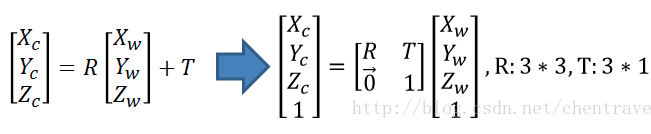## 2.相机坐标系->图像坐标系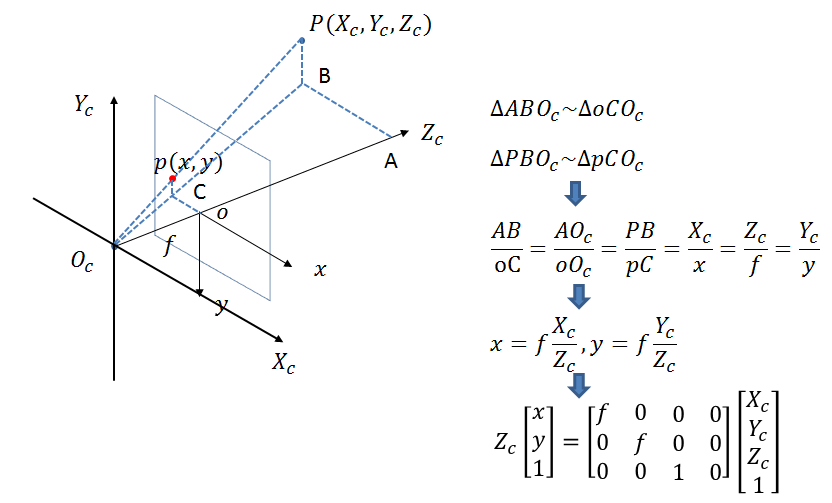此时投影点p的单位还是mm，并不是pixel，需要进一步转换到像素坐标系。

## 3.图像坐标系与像素坐标系

像素坐标系和图像坐标系都在成像平面上，只是各自的原点和度量单位不一样。图像坐标系的原点为相机光轴与成像平面的交点，通常情况下是成像平面的中点或者叫principal point。图像坐标系的单位是mm，属于物理单位，而像素坐标系的单位是pixel，我们平常描述一个像素点都是几行几列。所以这二者之间的转换如下：其中dx和dy表示每一列和每一行分别代表多少mm，即1pixel=dx mm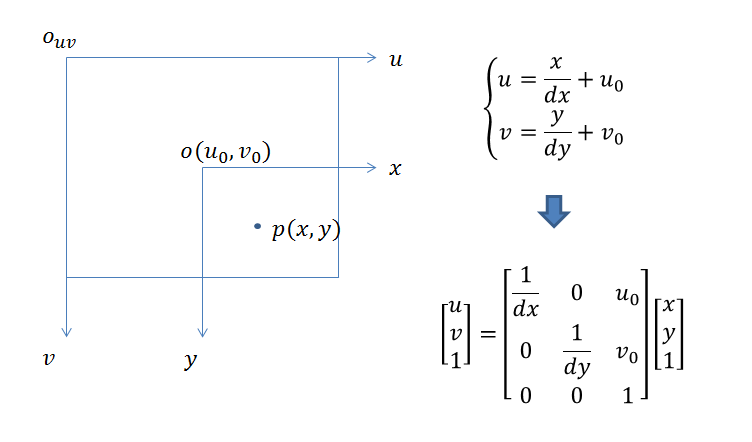那么通过上面四个坐标系的转换就可以得到一个点从世界坐标系如何转换到像素坐标系的。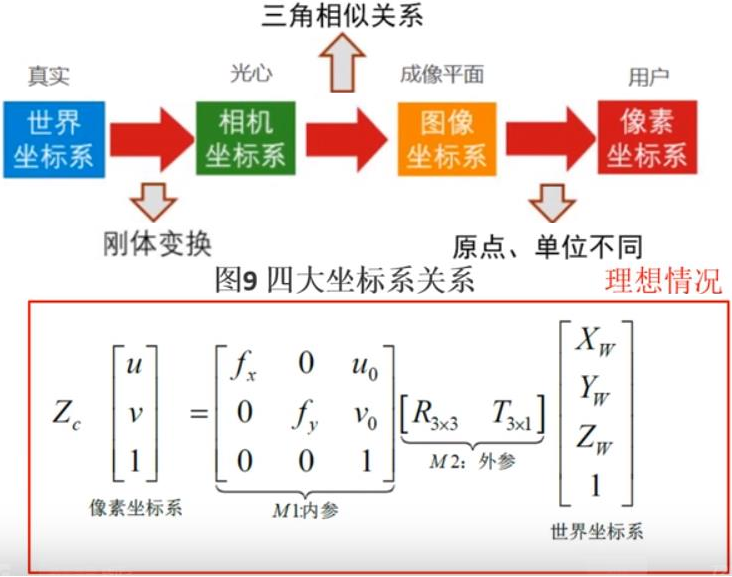python代码实现:

以下是实现变换的关键代码

相关可视化部分已经push到github:  https://github.com/PanJinquan/python-learning-notes

https://github.com/PanJinquan/python-learning-notes/blob/master/modules/utils_3d/camera_tools.py

# -*- coding: utf-8 -*-
"""
# --------------------------------------------------------
# @Project: Integral-Human-Pose-Regression-for-3D-Human-Pose-Estimation
# @Author : panjq
# @E-mail : pan_jinquan@163.com
# @Date   : 2020-02-04 16:03:01
# --------------------------------------------------------
"""
import sys
import os

sys.path.append(os.getcwd())

import cv2
import numpy as np
from modules.utils_3d import vis_3d as vis
from utils import image_processing

human36m_camera_intrinsic = {
# R，旋转矩阵
"R": [[-0.91536173, 0.40180837, 0.02574754],
[0.05154812, 0.18037357, -0.98224649],
[-0.39931903, -0.89778361, -0.18581953]],
# t，平移向量
"T": [1841.10702775, 4955.28462345, 1563.4453959],
# 焦距，f/dx, f/dy
"f": [1145.04940459, 1143.78109572],
# principal point，主点，主轴与像平面的交点
"c": [512.54150496, 515.45148698]

}

kinect2_camera_intrinsic = {

# R，旋转矩阵
"R": [[0.999853, -0.00340388, 0.0167495],
[0.00300206, 0.999708, 0.0239986],
[-0.0168257, -0.0239459, 0.999571]],
# t，平移向量
"T": [15.2562, 70.2212, -10.9926],
# 焦距，f/dx, f/dy
"f": [367.535, 367.535],
# principal point，主点，主轴与像平面的交点
"c": [260.166, 205.197]

}

camera_intrinsic = human36m_camera_intrinsic
# camera_intrinsic = kinect2_camera_intrinsic

class CameraTools(object):

@staticmethod
def convert_wc_to_cc(joint_world):
"""
世界坐标系 -> 相机坐标系: R * (pt - T):
joint_cam = np.dot(R, (joint_world - T).T).T
:return:
"""
joint_world = np.asarray(joint_world)
R = np.asarray(camera_intrinsic["R"])
T = np.asarray(camera_intrinsic["T"])
joint_num = len(joint_world)
# 世界坐标系 -> 相机坐标系
# [R|t] world coords -> camera coords
# joint_cam = np.zeros((joint_num, 3))  # joint camera
# for i in range(joint_num):  # joint i
#     joint_cam[i] = np.dot(R, joint_world[i] - T)  # R * (pt - T)
# .T is 转置, T is translation mat
joint_cam = np.dot(R, (joint_world - T).T).T  # R * (pt - T)
return joint_cam

@staticmethod
def convert_cc_to_wc(joint_world):
"""
相机坐标系 -> 世界坐标系: inv(R) * pt +T
joint_cam = np.dot(inv(R), joint_world.T)+T
:return:
"""
joint_world = np.asarray(joint_world)
R = np.asarray(camera_intrinsic["R"])
T = np.asarray(camera_intrinsic["T"])
# 相机坐标系 -> 世界坐标系
joint_cam = np.dot(np.linalg.inv(R), joint_world.T).T + T
return joint_cam

@staticmethod
def __cam2pixel(cam_coord, f, c):
"""
相机坐标系 -> 像素坐标系: (f / dx) * (X / Z) = f * (X / Z) / dx
cx,ppx=260.166; cy,ppy=205.197; fx=367.535; fy=367.535
将从3D(X,Y,Z)映射到2D像素坐标P(u,v)计算公式为：
u = X * fx / Z + cx
v = Y * fy / Z + cy
D(v,u) = Z / Alpha
=====================================================
camera_matrix = [[428.30114, 0.,   316.41648],
[   0.,    427.00564, 218.34591],
[   0.,      0.,    1.]])

fx = camera_intrinsic[0, 0]
fy = camera_intrinsic[1, 1]
cx = camera_intrinsic[0, 2]
cy = camera_intrinsic[1, 2]
=====================================================
:param cam_coord:
:param f: [fx,fy]
:param c: [cx,cy]
:return:
"""
# 等价于：(f / dx) * (X / Z) = f * (X / Z) / dx
# 三角变换， / dx, + center_x
u = cam_coord[..., 0] / cam_coord[..., 2] * f + c
v = cam_coord[..., 1] / cam_coord[..., 2] * f + c
d = cam_coord[..., 2]
return u, v, d

@staticmethod
def convert_cc_to_ic(joint_cam):
"""
相机坐标系 -> 像素坐标系
:param joint_cam:
:return:
"""
# 相机坐标系 -> 像素坐标系，并 get relative depth
# Subtract center depth
# 选择 Pelvis骨盆 所在位置作为相机中心，后面用之求relative depth
root_idx = 0
center_cam = joint_cam[root_idx]  # (x,y,z) mm
joint_num = len(joint_cam)
f = camera_intrinsic["f"]
c = camera_intrinsic["c"]
# joint image_dict，像素坐标系，Depth 为相对深度 mm
joint_img = np.zeros((joint_num, 3))
joint_img[:, 0], joint_img[:, 1], joint_img[:, 2] = CameraTools.__cam2pixel(joint_cam, f, c)  # x,y
joint_img[:, 2] = joint_img[:, 2] - center_cam  # z
return joint_img

def demo_for_human36m():
joint_world = [[-91.679, 154.404, 907.261],
[-223.23566, 163.80551, 890.5342],
[-188.4703, 14.077106, 475.1688],
[-261.84055, 186.55286, 61.438915],
[39.877888, 145.00247, 923.98785],
[-11.675994, 160.89919, 484.39148],
[-51.550297, 220.14624, 35.834396],
[-132.34781, 215.73018, 1128.8396],
[-97.1674, 202.34435, 1383.1466],
[-112.97073, 127.96946, 1477.4457],
[-120.03289, 190.96477, 1573.4],
[25.895456, 192.35947, 1296.1571],
[107.10581, 116.050285, 1040.5062],
[129.8381, -48.024918, 850.94806],
[-230.36955, 203.17923, 1311.9639],
[-315.40536, 164.55284, 1049.1747],
[-350.77136, 43.442127, 831.3473],
[-102.237045, 197.76935, 1304.0605]]
joint_world = np.asarray(joint_world)
# 关节点连接线
kps_lines = ((0, 7), (7, 8), (8, 9), (9, 10), (8, 11), (11, 12), (12, 13), (8, 14), (14, 15),
(15, 16), (0, 1), (1, 2), (2, 3), (0, 4), (4, 5), (5, 6))
# show in 世界坐标系
vis.vis_3d(joint_world, kps_lines, coordinate="WC", title="WC", set_lim=True, isshow=True)

kp_vis = CameraTools()

# show in 相机坐标系
joint_cam = kp_vis.convert_wc_to_cc(joint_world)
vis.vis_3d(joint_cam, kps_lines, coordinate="CC", title="CC", set_lim=True, isshow=True)
joint_img = kp_vis.convert_cc_to_ic(joint_cam)

joint_world1 = kp_vis.convert_cc_to_wc(joint_cam)
vis.vis_3d(joint_world1, kps_lines, coordinate="WC", title="WC", set_lim=True, isshow=True)

# show in 像素坐标系
kpt_2d = joint_img[:, 0:2]
image_path = "./data/s_01_act_02_subact_01_ca_02_000001.jpg"
image = image_processing.draw_key_point_in_image(image, key_points=[kpt_2d], pointline=kps_lines)
image_processing.cv_show_image("image_dict", image)

if __name__ == "__main__":
demo_for_human36m()


效果: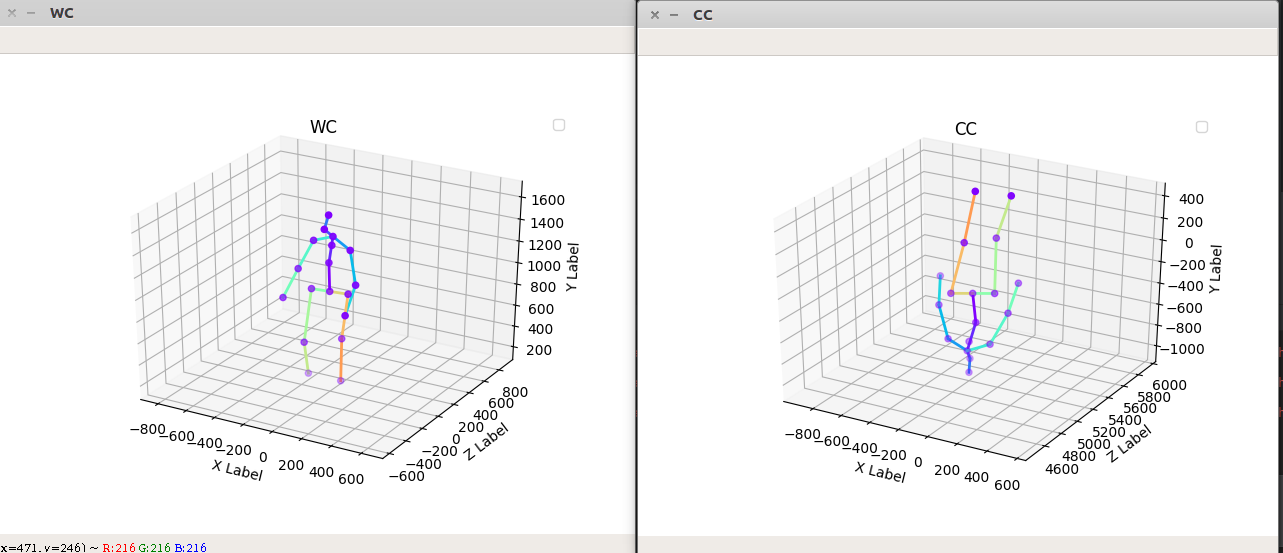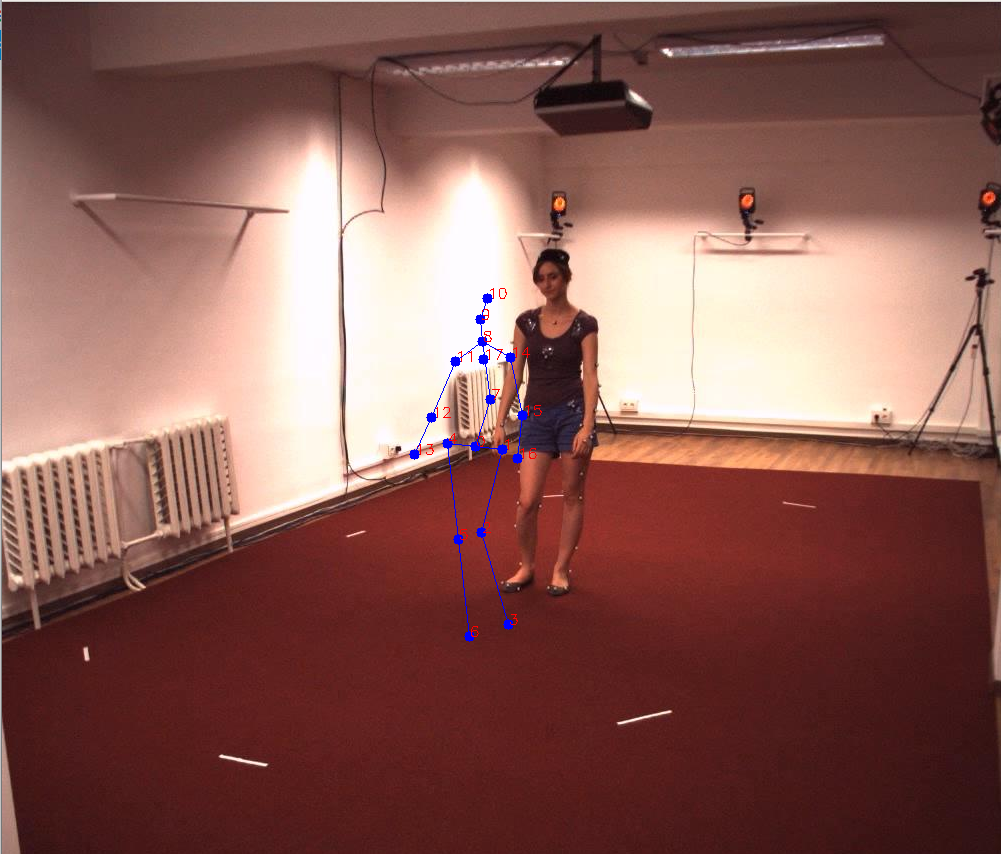展开全文世界坐标系 图像坐标系
• 世界坐标系,相机坐标系和图像坐标系的转换(Python)1.世界坐标->相机坐标2.相机坐标系->图像坐标系此时投影点p的单位还是mm，并不是pixel，需要进一步转换到像素坐标系。3.图像坐标系与像素坐标系像素坐标系和...
• 在涉及计算机视觉的许多领域都离不开世界坐标系、相机坐标系、图像坐标系以及像素坐标系,只有理解了这些才能对获取的图像进行准确的分析。由于摄像机可安放在环境中的任意位置，在环境中选择一个基准坐标系来描述...计算机视觉 图像处理
• 图像处理、立体视觉等等方向常常涉及到四个坐标系：世界坐标系、相机坐标系、图像坐标系、像素坐标系 构建世界坐标系只是为了更好的描述相机的位置在哪里，在双目视觉中一般将世界坐标系原点定在左相机或者右相机...
• 关于世界坐标系 相机坐标系 成像平面的一个很好的解释 同时也是对相机成像模型的一个解释
• 新学期第一天开始写的这篇文章，看看我啥时候能把他发...相机焦距f，相机之间距离为b，右上角为目标位置P（x,y），目标的水平坐标为x，相机离目标垂直距离（所求目标距离相机的深度）为z。 如果要计算深度z，必须.计算机视觉 人工智能
• 目录一、各坐标系简要介绍二、坐标系转换2.1 世界坐标系转为相机坐标系2.2 相机坐标系转为物理图像坐标系2.3 物理图像坐标系转为图像坐标系2.4 总结2.5 为什么要使用齐次坐标参考文献 一、各坐标系简要介绍 首先...计算机视觉 人工智能
• 本篇文章为对焦系列文章的第一篇：主要讲解UI坐标系和相机坐标系。其他相关文章如下： 【Android Camera1】Camera1 对焦(二) 对焦区域计算的几种方式 【Android Camera1】Camera1 对焦(三) 对焦标准化转换流程 ...Camera1 Android
•      ...计算机视觉领域中常见的四个坐标系：像素坐标系、图像坐标系，相机坐标系，世界坐标系。 像素坐标系（u,v）（u,v）（u,v） 图像坐标系（x,y）（x,y）（x,y） 相计算机视觉
• 三、世界坐标系转（Ow）化为相机坐标系（Oc） 四、相机坐标系（Oc）与图像坐标系（Ox-y） 五、图像坐标系（Ox-y）与像素坐标系（Ou-v） 问题2(1)：图像坐标系mm怎么转换为piex，即怎么计算成像平面中的1piex = ...
•计算机视觉 opencv
• 1.我做了手眼标定之后，得到了坐标从相机坐标系到机械臂基坐标系的转换方法，但是如何得到坐标从像素坐标系到相机坐标系的转换关系，这样我就可以将坐标从像素坐标系转换到机器人基坐标系，就可以引导机器人抓取目标...python
• 需完成RGB上的UVZ，转为IR坐标系下的UVZ 实现流程： 代码： import cv2 import os import numpy as np import json import matplotlib.pyplot as plt from PIL import Image, ImageTk,ImageDraw depth_f =[492.90python 计算机视觉 深度学习
• 相机坐标系(camera coordinate system)：在相机上建立的坐标系，为了从相机的角度描述物体位置而定义，作为沟通世界坐标系和图像/像素坐标系的中间一环。单位为m。 图像坐标系(image coordinate...
• 这个过程中会涉及到四个坐标系，即世界坐标系、相机坐标系、图像坐标系、像素坐标系。下面将详细介绍四个坐标系的函已并且记录其的转换过程。 图像中所涉及的四个坐标系及其含义： Ow - Xw - Yw - Zw ：世界坐标...
• 物体再空间中的坐标，说白了就是除了相机坐标系外（其实相等也没关系）的另一个坐标系，可以以空间任意一个点建立坐标系 相机坐标系 相机的成像是位于感光元件上，可以想象再往里存在一个相机的原点，以它建立...计算机视觉
• 一、四个坐标系简介和转换 相机模型为以后一切标定算法的关键，只有这边有相当透彻的理解，对以后的标定算法才能有更好的理解。...我觉得首先我们要理解相机模型中的四个平面坐标系的关系：像素平面坐标系（u,v...
• 按照十四讲的顺序我们首先接触的是像素坐标系、图像坐标系、相机坐标系、相机归一化坐标系、世界坐标系，这里学着学着就蒙了，所以我建议先学习投影模型，我认为二者可以理解为互逆过程。从零开始学习SLAM
• 图像坐标系、相机坐标系和世界坐标系的定义，及三者之间的变换关系。 图像坐标系 1.图像像素坐标系 原点：图像左上角P0点 单位：像素 横坐标u：图像数组中的列数 纵坐标v：图像数组中的行数 2.图像物理坐标系 在...图像坐标系 世界坐标系
• 最近在网上看到了很多关于坐标系转换的帖子，但是其内容很多都是相互转载（甚至还有一部分是错误的），同时大部分的文章内容都是告诉你四种坐标系间的相互转化的数学公式，看完之后很多时候还是不知所云，本文意在...图像物理坐标系 世界坐标系
• 由于最近在学习关于相机标定的知识，所以了解大量相机知识，但通过大量资料学习后发现，网上很多对坐标系的转化过程无明确过程，使得数学基础不好的笔者十分苦恼，由此整理了一篇关于坐标系转化的文章，仅供参考。...
• 世界坐标系、相机坐标系、图像坐标系、像素坐标系之间的转换 图像处理、立体视觉等方向常常涉及到四个坐标系：世界坐标系、相机坐标系、图像坐标系、像素坐标系。例如下图： 构建世界坐标系只是为了更好的描述...世界坐标系 图像坐标系
• 点击上方“小白学视觉”，选择加"星标"或“置顶”重磅干货，第一时间送达 本文转自：新机器视觉相机的成像过程涉及到四个坐标系：世界坐标系、相机坐标系、图像坐标系、像素坐标系...计算机视觉 人工智能 opencv python
• 机器视觉（六）——像素坐标系、图像坐标系、相机坐标系以及世界坐标系 目录1. 像素坐标系2. 图像坐标系3.相机坐标系4.世界坐标系 1. 像素坐标系 如下图所示：像素坐标系u-v的原点为O0， 横坐标u和纵坐标v分别是图像...计算机视觉 opencv...